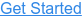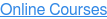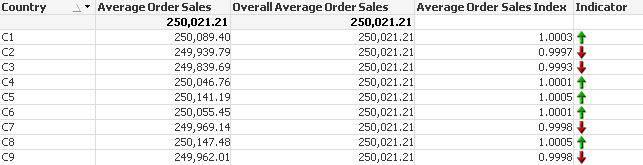Where do you want to go?# Performance (and other) benefits of using expression column and label references

by Mike Steedle, on Apr 23, 2013 11:45:45 PM

As has been documented elsewhere, there is not always a performance benefit from using column or label references in charts, but there are performance benefits when you really need them: resource intensive functions. Consider the following example, using the dreaded Aggr function.

Source data:

```Base:
LOAD 'C' & Left(RowNo(),1) as Country,
Left(RowNo(),4) as OrderID,
Round(Rand()*500) as Sales,
Round(Rand()*30) as UnitCost,
Round(Rand()*10) as Quantity
AUTOGENERATE 10000000;```

Output:Scenario expressions and calc times:

 Explicit Label Column Explicit (sloppy) Average Order Sales Avg(Aggr(Sum(Sales),OrderID)) Avg(Aggr(Sum(Sales),OrderID)) Avg(Aggr(Sum(Sales),OrderID)) Avg(Aggr(Sum(Sales),OrderID)) Overall Average Order Sales Avg(TOTAL Aggr(Sum(Sales),OrderID)) Avg(TOTAL Aggr(Sum(Sales),OrderID)) Avg(TOTAL Aggr(Sum(Sales),OrderID)) Avg(TOTAL Aggr(Sum(Sales),OrderID)) Average Order Sales Index Avg(Aggr(Sum(Sales),OrderID)) / Avg(TOTAL Aggr(Sum(Sales),OrderID)) [Average Order Sales] / [Overall Average Order Sales] Column(1) / Column(2) avg(Aggr(sum(Sales),OrderID)) / avg(TOTAL Aggr(sum(Sales),OrderID)) Indicator If( Avg(Aggr(Sum(Sales),OrderID)) / Avg(TOTAL Aggr(Sum(Sales),OrderID)) > 1, 'qmem:///BuiltIn/arrow_n_g.png', If( Avg(Aggr(Sum(Sales),OrderID)) / Avg(TOTAL Aggr(Sum(Sales),OrderID)) < 1, 'qmem:///BuiltIn/arrow_s_r.png' )) If( [Average Order Sales Index] > 1, 'qmem:///BuiltIn/arrow_n_g.png', If( [Average Order Sales Index] < 1, 'qmem:///BuiltIn/arrow_s_r.png' )) If( Column(3) > 1, 'qmem:///BuiltIn/arrow_n_g.png', If( Column(3) < 1, 'qmem:///BuiltIn/arrow_s_r.png' )) If( Avg( Aggr( Sum( Sales ) ,OrderID ) ) / Avg( TOTAL Aggr( Sum( Sales ) ,OrderID ) ) > 1, 'qmem:///BuiltIn/arrow_n_g.png', If( Avg( Aggr( Sum( Sales ) ,OrderID ) ) / Avg( TOTAL Aggr( Sum( Sales ) ,OrderID ) ) < 1, 'qmem:///BuiltIn/arrow_s_r.png' ) ) Calc Time (Init) 2059 1186 1154 3198 Calc Time (Filter) 1201 670 671 1841

Column and Label reference examples performed almost 2x as quickly as explicit formulas.

Note the other possible danger of using explicit expression formulas in the final column: writing an explicit formula slightly differently, like changing the case of a function or inserting spaces before or after parentheses, makes performance even worse (about 3x worse, in this example). QlikView doesn't detect that sum(Sales) and Sum(Sales) mean the same thing within a chart, even though they have the same result. With column or label references, inconsistency of expressions within a chart isn't a possibility. They also result in fewer expressions to maintain if fields or business rules change, and derived expressions are shorter and easier to understand.

In another example, I found a slight calculation time improvement with column and reference labels when I added a flag to the data model, then used IF statements to conditionally return the values where the flag was equal 1. That said, I found no performance improvement when using set analysis to accomplish the same end, e.g. {<Flag={1}>}. In no instances tested was the performance worse with column or label references than explicit expressions.

To summarize, the best case scenario is that using column or label references shortens calculation time and makes the chart expressions easier to understand and maintain. The worst case scenario is that using column or label references merely makes the chart expressions easier to understand and maintain. Thus, consistently using column or label references remains a good development practice. Just remember to Disable Drag and Drop in the chart if you are using column references in a table.

Topics:PerformanceQlikView